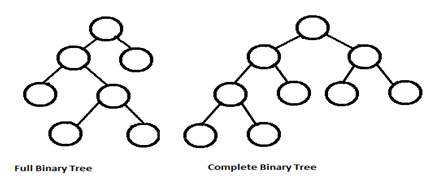# Complete Binary Tree depth - Data Structure

Q.  Which of the following is the depth of a Complete Binary Tree?
- Published on 26 Aug 15

a. Dn = n log2n
b. Dn = n log2n + 1
c. Dn = log2n
d. Dn = log2n + 1

ANSWER: Dn = log2n + 1

#### Discussion

• Nirja Shah   -Posted on 21 Nov 15
The depth of complete binary tree of n nodes will be Dn=log 2 (n+1). Here Dn is the height or depth of the tree and n is the number of nodes.
A complete binary tree is a binary tree where all the levels have maximum number of nodes except possibly the last level.
There is a difference between complete binary tree and full binary tree.
A binary tree is called as full binary in which every node other than the leaves has two children.
It is also called as 2-tree.## ➨ Post your comment / Share knowledgeEnter the code shown above:

(Note: If you cannot read the numbers in the above image, reload the page to generate a new one.)